# The volume of the different geometric shapes

Про матеріал
Whereas the basic formula for the area of a rectangular shape is length × width, the basic formula for volume is length × width × height.
Перегляд файлу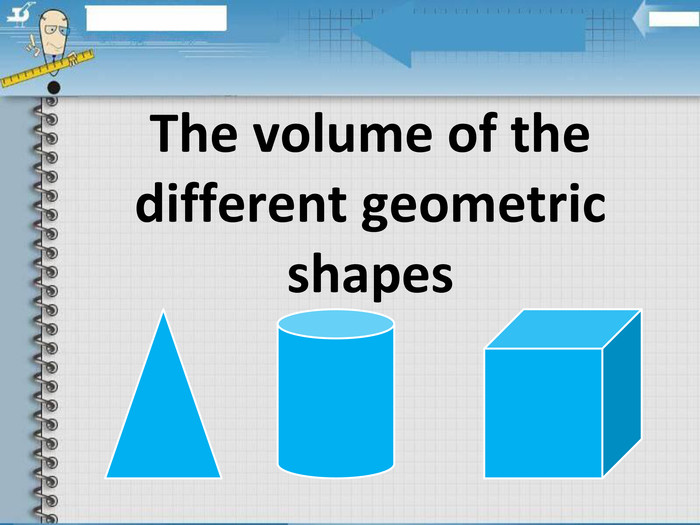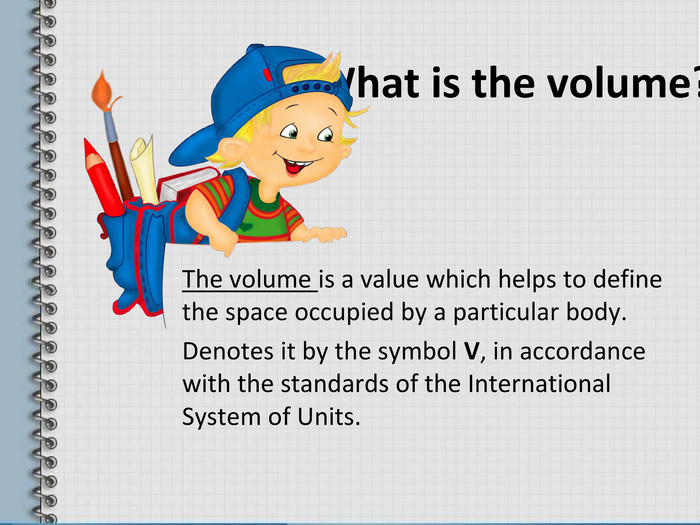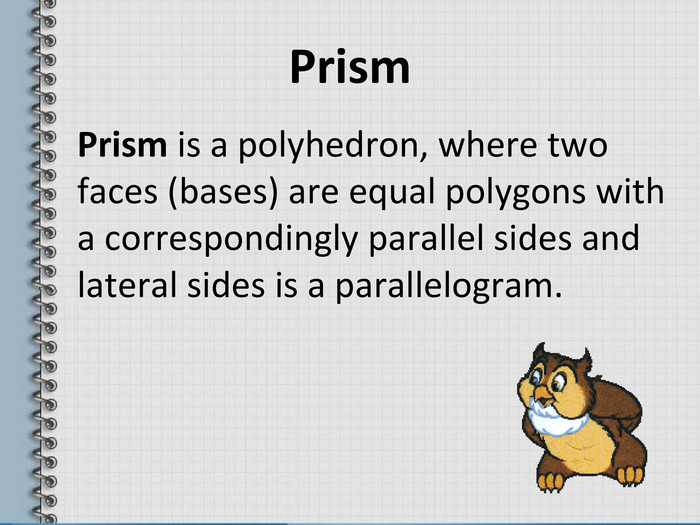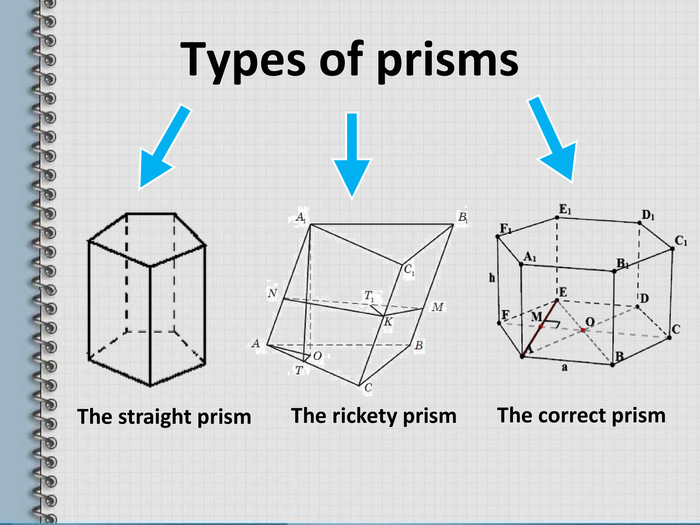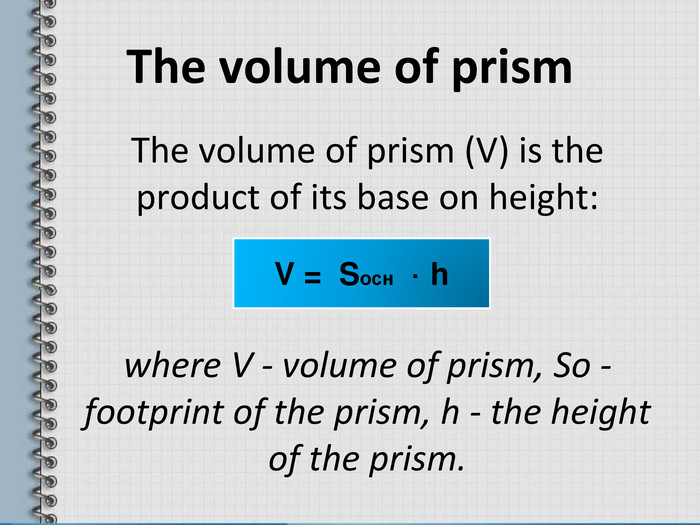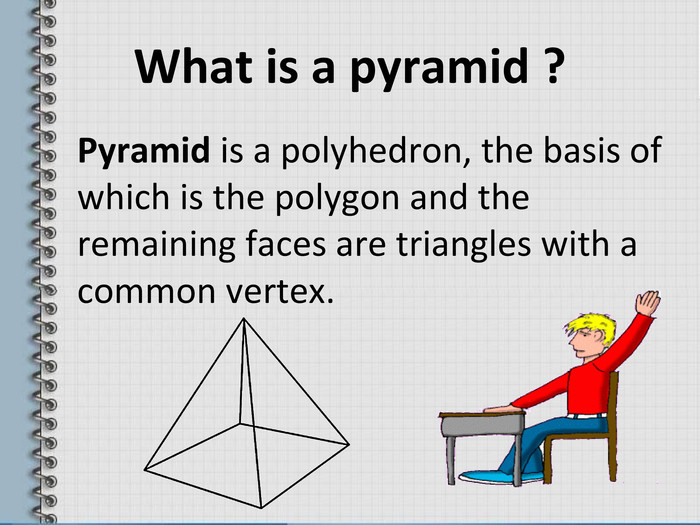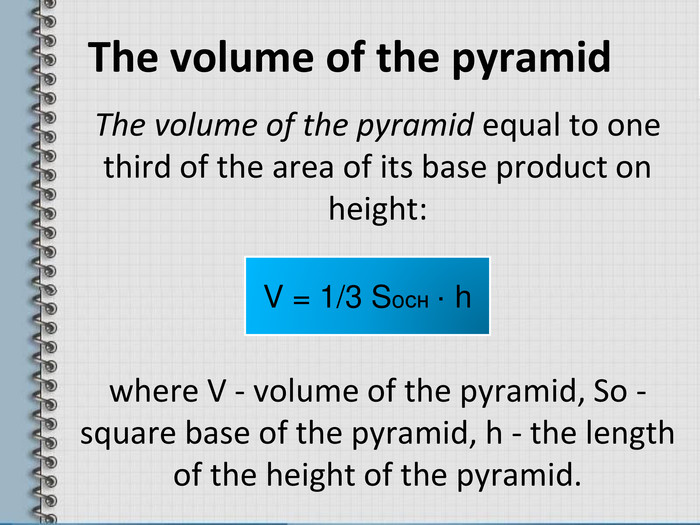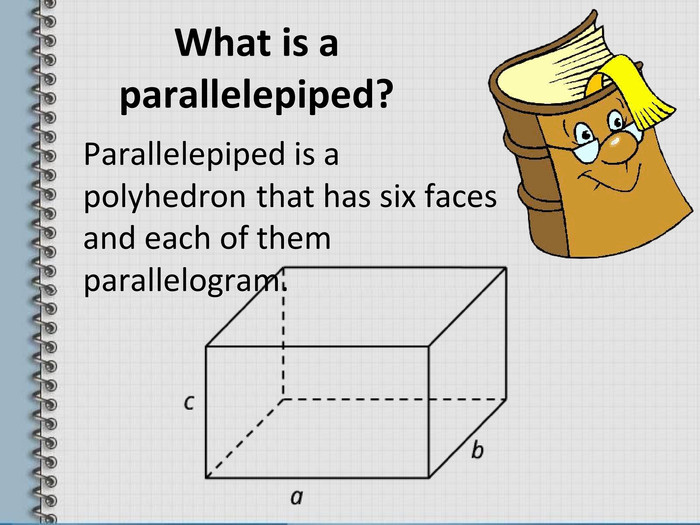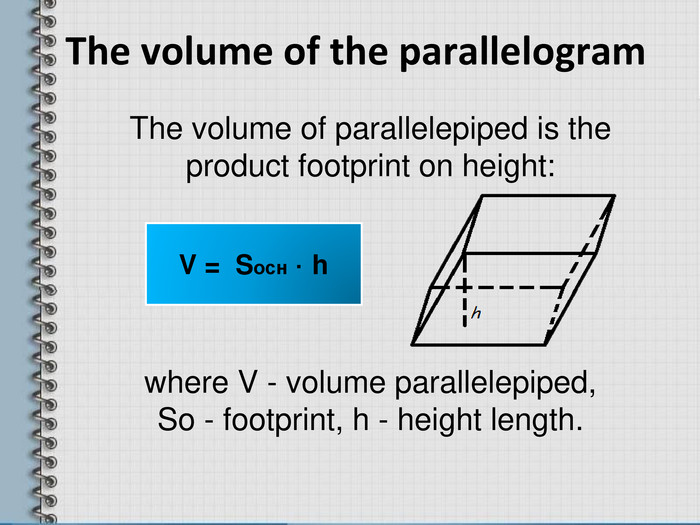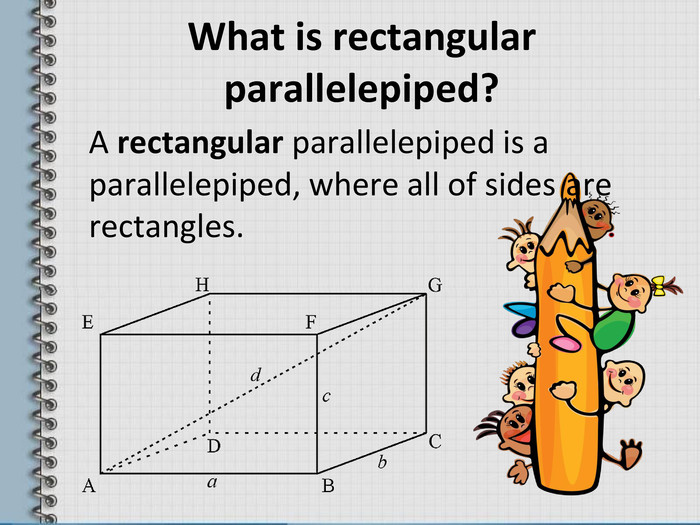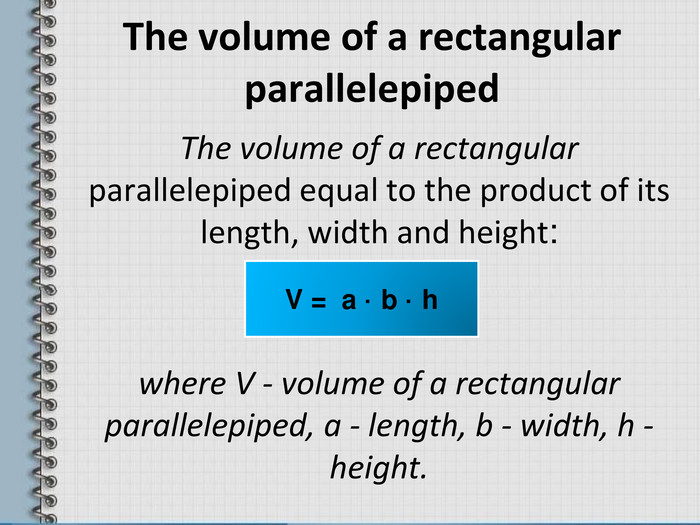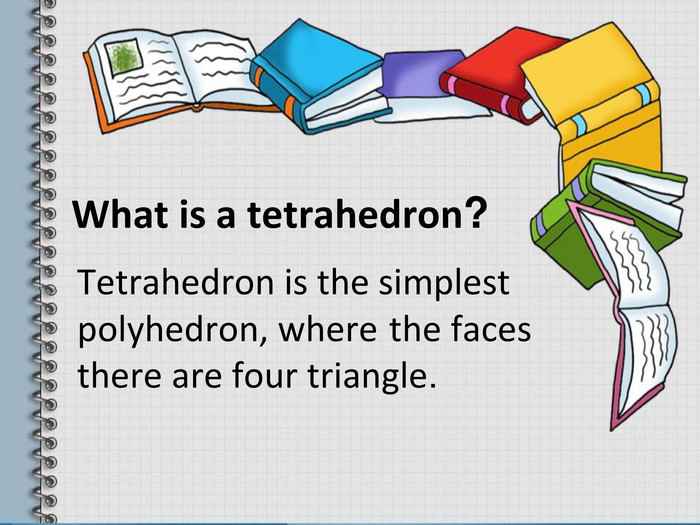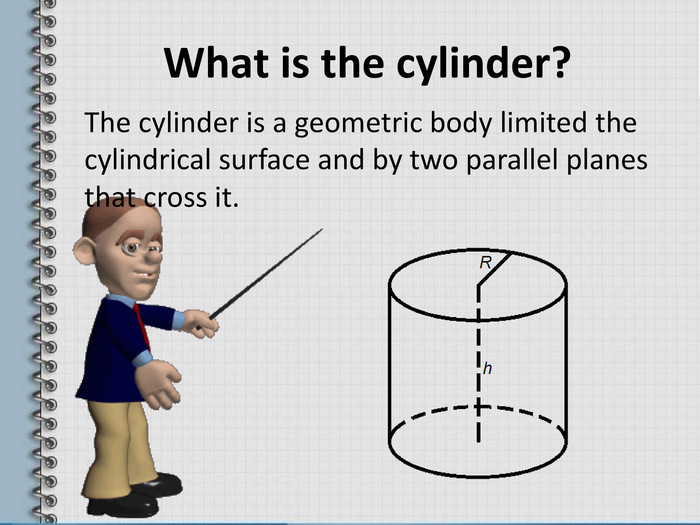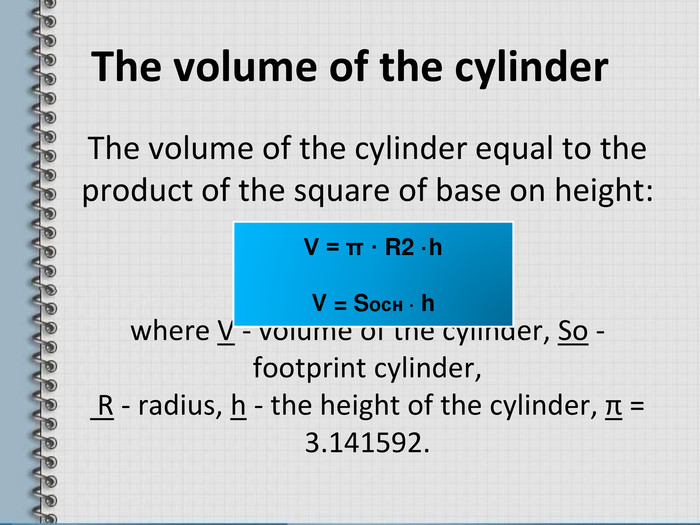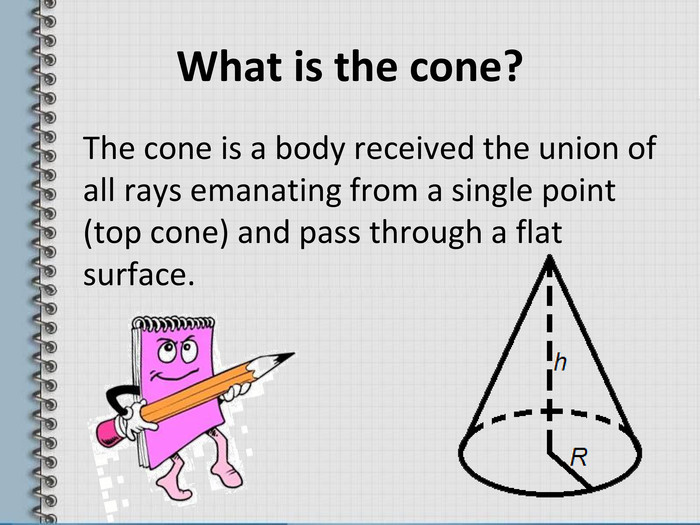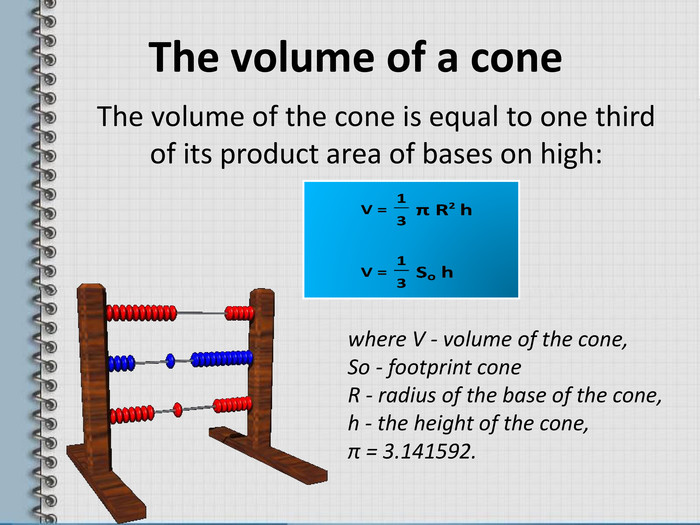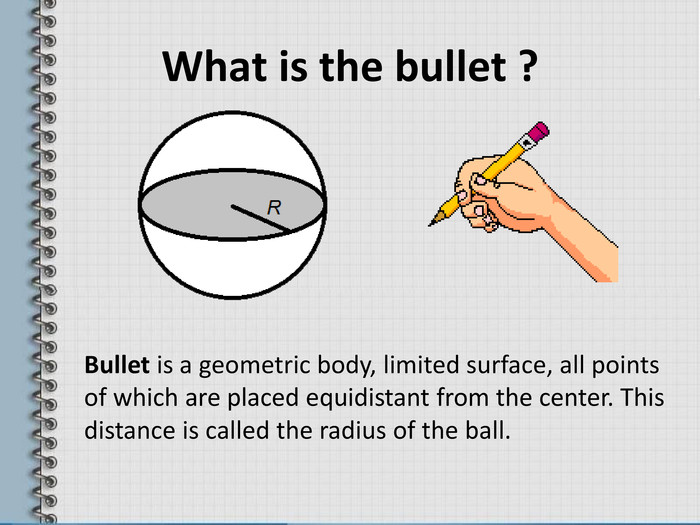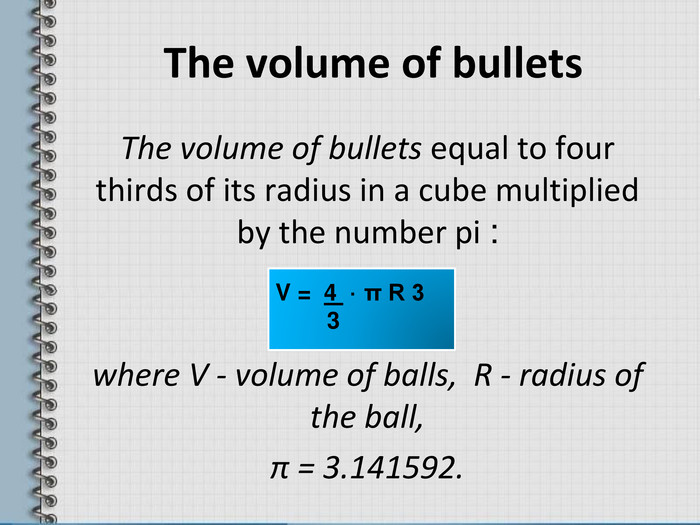Зміст слайдів
Номер слайду 1

The volume of the different geometric shapes

Номер слайду 2

What is the volume? The volume is a value which helps to define the space occupied by a particular body. Denotes it by the symbol V, in accordance with the standards of the International System of Units.

Номер слайду 3

Prism Prism is a polyhedron, where two faces (bases) are equal polygons with a correspondingly parallel sides and lateral sides is a parallelogram.

Номер слайду 4

Types of prisms The correct prism The straight prism The rickety prism

Номер слайду 5

The volume of prism The volume of prism (V) is the product of its base on height: where V - volume of prism, So - footprint of the prism, h - the height of the prism. V = Sосн · h

Номер слайду 6

What is a pyramid ? Pyramid is a polyhedron, the basis of which is the polygon and the remaining faces are triangles with a common vertex.

Номер слайду 7

The volume of the pyramid The volume of the pyramid equal to one third of the area of its base product on height: where V - volume of the pyramid, So - square base of the pyramid, h - the length of the height of the pyramid. V = 1/3 Soсн · h

Номер слайду 8

What is a parallelepiped? Parallelepiped is a polyhedron that has six faces and each of them parallelogram.

Номер слайду 9

The volume of the parallelogram The volume of parallelepiped is the product footprint on height: where V - volume parallelepiped, So - footprint, h - height length. V = Sосн · h

Номер слайду 10

What is rectangular parallelepiped? A rectangular parallelepiped is a parallelepiped, where all of sides are rectangles.

Номер слайду 11

The volume of a rectangular parallelepiped The volume of a rectangular parallelepiped equal to the product of its length, width and height: where V - volume of a rectangular parallelepiped, a - length, b - width, h - height.

Номер слайду 12

What is a tetrahedron? Tetrahedron is the simplest polyhedron, where the faces there are four triangle.

Номер слайду 13

The volume of a regular tetrahedron V = a3√2 12 where V - volume of a regular tetrahedron, a - the length of the edges of a regular tetrahedron.

Номер слайду 14

What is the cylinder? The cylinder is a geometric body limited the cylindrical surface and by two parallel planes that cross it.

Номер слайду 15

The volume of the cylinder The volume of the cylinder equal to the product of the square of base on height: where V - volume of the cylinder, So - footprint cylinder, R - radius, h - the height of the cylinder, π = 3.141592. V = π · R2 ·h V = Soсн · h

Номер слайду 16

What is the cone? The cone is a body received the union of all rays emanating from a single point (top cone) and pass through a flat surface.

Номер слайду 17

The volume of a cone The volume of the cone is equal to one third of its product area of bases on high: where V - volume of the cone, So - footprint cone R - radius of the base of the cone, h - the height of the cone, π = 3.141592.

Номер слайду 18

What is the bullet ? Bullet is a geometric body, limited surface, all points of which are placed equidistant from the center. This distance is called the radius of the ball.

Номер слайду 19

The volume of bullets The volume of bullets equal to four thirds of its radius in a cube multiplied by the number pi : where V - volume of balls, R - radius of the ball, π = 3.141592. V = 4 · π R 3 3

Номер слайду 20

Thank you for attention !

ppt
Додано
18 квітня
Переглядів
45
Оцінка розробки
Відгуки відсутні